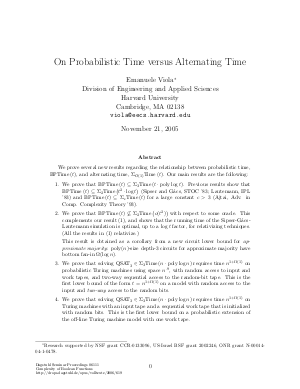Document# On Probabilistic Time versus Alternating Time

### Author Emanuele Viola## File

DagSemProc.06111.11.pdf
• Filesize: 305 kB
• 26 pages

## Cite As

Emanuele Viola. On Probabilistic Time versus Alternating Time. In Complexity of Boolean Functions. Dagstuhl Seminar Proceedings, Volume 6111, Schloss Dagstuhl - Leibniz-Zentrum für Informatik (2006)
https://doi.org/10.4230/DagSemProc.06111.11

## Abstract

Sipser and GÃƒÂ¡cs, and independently Lautemann, proved in '83 that probabilistic polynomial time is contained in the second level of the polynomial-time hierarchy, i.e. BPP is in Sigma_2 P. This is essentially the only non-trivial upper bound that we have on the power of probabilistic computation. More precisely, the Sipser-GÃƒÂ¡cs-Lautemann simulation shows that probabilistic time can be simulated deterministically, using two quantifiers, **with a quadratic blow-up in the running time**. That is, BPTime(t) is contained in Sigma_2 Time(t^2). In this talk we discuss whether this quadratic blow-up in the running time is necessary. We show that the quadratic blow-up is indeed necessary for black-box simulations that use two quantifiers, such as those of Sipser, GÃƒÂ¡cs, and Lautemann. To obtain this result, we prove a new circuit lower bound for computing **approximate majority**, i.e. computing the majority of a given bit-string whose fraction of 1's is bounded away from 1/2 (by a constant): We show that small depth-3 circuits for approximate majority must have bottom fan-in Omega(log n). On the positive side, we obtain that probabilistic time can be simulated deterministically, using three quantifiers, in quasilinear time. That is, BPTime(t) is contained in Sigma_3 Time(t polylog t). Along the way, we show that approximate majority can be computed by uniform polynomial-size depth-3 circuits. This is a uniform version of a striking result by Ajtai that gives *non-uniform* polynomial-size depth-3 circuits for approximate majority. If time permits, we will discuss some applications of our results to proving lower bounds on randomized Turing machines.
##### Keywords
• Probabilistic time
• alternating time
• polynomial-time hierarchy
• approximate majority
• constant-depth circuit

## Metrics

• Access Statistics
• Total Accesses (updated on a weekly basis)
0